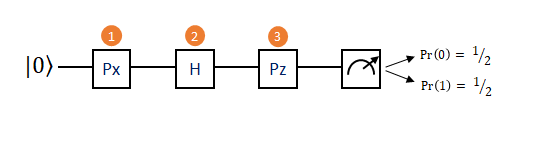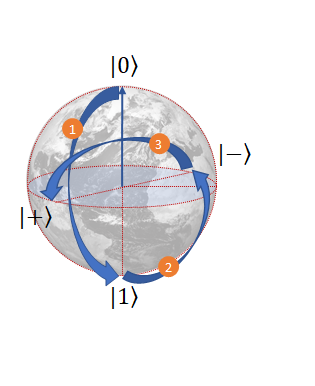Seleccionar página

Two weeks ago we familiarised ourselves with the fundamental concepts of quantum computing. In this article, we continue building up more intuition about what a computation is, and what it looks like. At QWA we believe in the power of analogies, so let us start by introducing Travis, an avid traveller who wants to see every place on Earth!

Travis’s best friend, Scott, has decided to help him make his dream become a reality. But Scott wants to play a little game with Travis first. Keep in mind that Scott is a computer scientist with a passion for quantum physics, so expect the rest of the story to be slightly unusual. Scott will indeed buy Travis a plane ticket, but the destinations are going to be constrained by an initial choice. “Travis, would you prefer a classical Earth ticket or a quantum Earth ticket?” Scott asks his friend. Travis, who is busy packing, replies absently “Ehm, sure, classical will do”. Scott giggles and hands Travis the chosen ticket.

At the airport, Travis speaks to the airline representative who, to his great surprise, announces that the only possible flights for him to choose are to the North or South Pole; “What is happening here!?” shrieks Travis in disbelief!

Scott, who is a good friend after all, knew this would happen. He makes a surprise appearance at the airport just as Travis is resigned to giving up his traveller’s dreams, and hands him the quantum Earth ticket: “With this, my friend, you will be able to travel everywhere on the planet!”.

This is the core of the analogy between a classical bit and a quantum bit, a qubit. Classical bits can only be in one of two possible states, “zero” or “one”, just like Travis is stuck either at the North or South pole on the classical Earth. Qubits, on the other hand, can access a much larger space: all points on the surface of a sphere. On the quantum Earth, Travis is free to visit anywhere on the planet’s surface he chooses. In quantum information theory, the “Earth” of the qubit is known as Bloch sphere, named after the physicist Felix Bloch. Meanwhile, the arrow that points from the centre of the quantum Earth to Travis’s position on the surface is known as the Bloch vector.

Having the sphere model clear in mind, this is now the right time to clarify the concept of superposition, introduced in our previous entries. If the North and the South Poles are our reference basis, then when Travis is anywhere else on Earth he will be in a combination of north and south. The usual coordinate system used by humans to indicate the departure from the poles is composed of latitude and longitude. The same coordinates can be used for qubits! When the qubit state is not a zero (North Pole) or a one (South Pole), it means the state is in a superposition of the two, captured by a non-zero (and non 180°) value of the latitude. If the state sits at the equator of the sphere, then it is in an equal superposition of north and south, of zero and one.

One should think of superposition as a probability distribution. The great difference between a conventional probability distribution and quantum mechanical superpositions, is that the “amount” of superposition — i.e. how much “zero” and “one” — is not specified by positive real numbers, but by complex — in the mathematical sense — amplitudes. In particular, amplitudes can be negative, which opens up the possibility of interference: positive and negative amplitudes can combine to suppress a particular outcome, very much like the crests and troughs of a water wave can partially or totally cancel each other out. The power of interference in quantum computation was wonderfully explained by Scott Aaronson and Zach Weinersmith in the comic SMBC.

Representing a qubit state as a point on a sphere also helps to understand, and to easily visualise, the concept of quantum gate operations on qubits. For simplicity, let’s assume Travis prefers to holiday on the surface of the Earth, like the rest of us, and doesn’t venture into the interior. In this case, he can always make it back home safely, by simply undoing the trip he makes initially. In the language of quantum mechanics, such reversible operations are known as unitary operations, and are nothing but rotations of the Bloch vector on the surface of the Bloch sphere.

It turns out you can build up any rotation you like — and thus get anywhere you please on the surface of the Bloch sphere — by a suitable sequence of rotations around the three main axes (X,Y,Z) of 3D space. Physicists and mathematicians use a special name for the matrices that represent 180° rotations around the main axes, i.e. rotations which take a point to its opposite (antipode) on the sphere’s surface, for instance North to South Pole. These are known as the Pauli matrices (Pauli-X, Pauli-Y, and Pauli-Z). Unexpectedly, the name comes from Wolfgang Pauli, one of the early pioneers of quantum physics (read more about the legend of his curse here). Because of their simple, and yet fundamental significance, the Pauli matrices occupy a very special place in the theory of quantum computation.

In the previous Medium article, we played with the idea of quantum computing as a musical symphony. Here we are going to push that idea a little further, by introducing the music score where magic happens. A computation, in its most intuitive form, is a process where an input is transformed into an output state, by means of well defined operations. The input is the question of the computation, for instance “What is the result of 34^23?”, “Find the first name of Prof. Schroedinger in this database”, “Is 28493 a prime number?”, the output is the answer “1.675e+35”, “Erwin”, “Yes, it is a prime number”. The set of operations in between, for which a better term in this context is gates, is what algorithms are made of. This general scheme applies both to classical and quantum computations. But, as the great physicist James Clerk Maxwell would have (probably) said, the demon is in the details.

For one qubit, the score is a single wire. Just like in music, time goes from left to right, and at the very beginning we append the initial state of the qubit. Conventionally, this is zero, written using the standard bra-ket notation |0>.

The score needs to be populated. The elements that live on the line are single-qubit gates, simple rotations, which transform the state according to their meaning. At the end of the score, if we want to have a classical result from the computation, we need to perform a measurement. A measurement is a non-reversible process, which outputs a single bit of information for each qubit. The probabilities of obtaining a zero or a one depend on the amplitudes of the superposition. A simple example of a quantum circuit for one qubit is shown below.A simple circuit for one qubit: Time goes from left to right, so a Pauli-X is the first gate to be applied.

While we met the Pauli matrices before, the gate H is a new entry. H stands for Hadamard gate, and its role is akin to a Fourier transform: It projects the standard basis elements (North-South) to a new basis on the equator of the sphere (East-West). Mathematically, it corresponds to a combination of two rotations, around the X and Y axis respectively. At the end of the simple circuit above we end up on the equator, and therefore the probabilities of observing a zero or a one are both 50%, or simply ½.The Bloch sphere representation of the circuit above. The numbers indicate the corresponding gates.

The same ideas apply to a computation performed on a multi-qubit input state. One of the most powerful results in quantum computing says that any multi-qubit unitary operation can be arbitrarily approximated by a finite sequence of one- and two-qubit gates. This is captured by the concept of universal gate set. A set of gates is universal if any operation on a quantum computer can be reduced to some combination of those gates. Universal sets need not to be large. In fact, three gates will suffice: the Hadamard gate, a single qubit 45° rotation around the Z-axis of the Bloch sphere, and the CNOT gate, a two-qubit entangling gate.

The CNOT gate is a controlled gate: it acts on a target qubit depending on the state of a control qubit. If the state of the control qubit is |1>, then it performs a Pauli-X rotation on its target. Attentive readers will object, “well you can actually do the same with classical bits, just replace the Pauli-X with a bit flip, where is the trick here?”. Fair objection! The answer is, the trick lies with superposition.

Classically, the control bit can only be in exactly one state, either zero or one. The truth table, a table which lists all possible outcomes of a logical gate, for the controlled-not classical gate is then the following:

The truth table for quantum states is equivalent, but superposition allows for more fun outcomes. If the control state is at the equator of the sphere, i.e. an equal superposition of |0> and |1>, and the target is in a simple |0>, then the following happens:

Because the control qubit is in a superposition state, the target qubit undergoes a flip in one branch of the computation, but not on the other. In a way, we are computing two rows of the classical truth table, by applying only a single quantum gate. The resulting state is an entangled state commonly known as a Bell state (you guessed it right! Also named after a great physicist — John Stewart Bell), which is represented mathematically as 0.707(|0>|0> + |1>|1>).

To summarise, any quantum computation can be modelled as a sequence of single-qubit rotations and CNOT gates. For sure, this is not the only way to compute on a quantum computer, but it is perhaps the simplest to understand — because of its resemblance to classical computation — and to visualise. The music score model is known as the circuit model, and the most common pattern is input state → gates → output state → measurements → classical outcomes.

For completeness, let us emphasise that the circuit model captures the essence of digital quantum computation, where information is processed by means of a discrete sequence of gates. There exist also proposals for analog, i.e. continuous, quantum computation. Quantum annealing is a particular framework for analog quantum computing, whose aim is to tackle combinatorial optimisation problems. We will discuss the physics and promises of quantum annealers, machines designed to find the minimum of a given target function, in a future entry. For more than a decade scientists have been discussing the merits of quantum annealers over classical computers; interested readers hungry for the details now can find a recent technical article, on the readiness of quantum annealers for industrial problems, here.

Today, it is possible to access rudimental quantum processors over the internet. The IBM Quantum Experience has perhaps the most intuitive platform to experiment with quantum circuits. It offers a user-friendly GUI, where gates can be dragged-and-dropped on the quantum circuit’s lines. An instance of Grover’s search algorithm on two qubits is shown below.